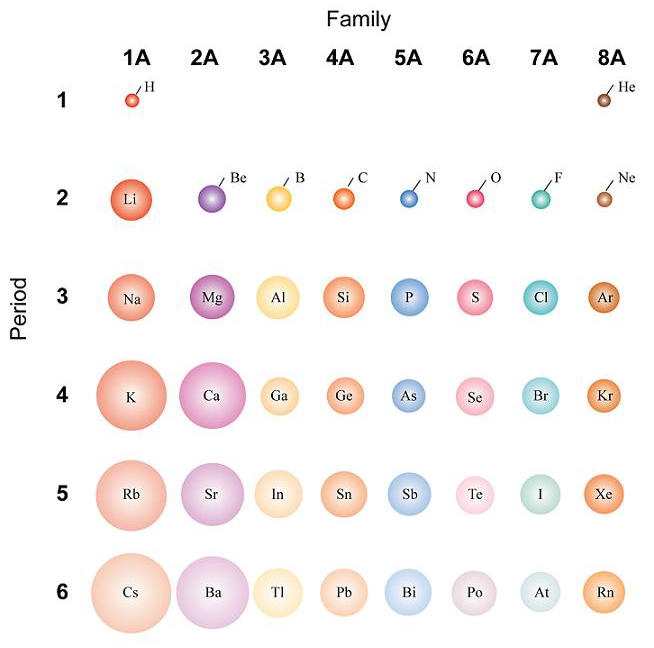## Atomic Size

#### Learning Objective

• Discuss the trend of atomic size in the periodic table.

#### Key Points

• Atomic radii vary predictably across the periodic table. For instance, the radii generally decrease along each period (row) of the table from left to right and increase down each group (column).
• As the atomic number increases along each row of the periodic table, the additional electrons go into the same outermost shell, causing the atomic radius to decrease due to the increasing nuclear charge.
• When moving down a group of the periodic table, the atomic radius increases because of the presence of additional principal energy levels, which are further away from the nucleus.

#### Terms

• periodA horizontal row in the periodic table, which signifies the total number of electron shells in an element’s atom.
• noble gasAny of the elements of group 18 of the periodic table, being monoatomic and (with very limited exceptions) inert.
• atomic radiusA measure of the size of an atom. Assuming atoms have a spherical shape, the radius of the sphere describes the size of the atom.

The atomic radius of a chemical element is a measure of the size of its atoms. It represents the mean distance from the nucleus to the boundary of the surrounding cloud of electrons.

Atomic radii vary in a predictable manner across the periodic table. Radii generally decrease along each period (row) of the table from left to right and increase down each group (column). These trends in atomic radii (as well as trends in various other chemical and physical properties of the elements) can be explained by considering the structure of the atom.Atomic Sizes (Radii)The atomic size trends across a period and down a group (‘family’ in this figure) of the periodic table are shown in this figure.

## Moving Across the Periodic Table

As the atomic number increases along each row of the periodic table, the additional electrons go into the same outermost principal energy level (also known as valence level). This can be predicted to lead to

1. an increase in atomic size because of additional repulsions between electrons,
2. a decrease in size because of the additional protons in the nucleus,
3. no effect at all as the two opposing tendencies of electron repulsion and nuclear attraction balance each other out.

Experiments have shown that the first case is what happens: the increase in nuclear charge overcomes the repulsion between the additional electrons in the valence level. Therefore, the size of atoms decreases as one moves across a period from left to right in the periodic table.

## Moving Down the Periodic Table

The principal energy levels hold electrons at increasing radii from the nucleus. In a noble gas, the outermost level is completely filled; therefore, the additional electron that the following alkali metal (Group I) possesses will go into the next principal energy level, accounting for the increase in the atomic radius. Therefore, atomic size, or radius, increases as one moves down a group in the periodic table.